Theory and Modern Applications

# Homomorphisms and derivations in ${C}^{\ast }$-ternary algebras via fixed point method

## Abstract

Park (J. Math. Phys. 47:103512, 2006) proved the Hyers-Ulam stability of homomorphisms in ${C}^{\ast }$-ternary algebras and of derivations on ${C}^{\ast }$-ternary algebras for the following generalized Cauchy-Jensen additive mapping:

$2f\left(\frac{{\sum }_{j=1}^{p}{x}_{j}}{2}+\sum _{j=1}^{d}{y}_{j}\right)=\sum _{j=1}^{p}f\left({x}_{j}\right)+2\sum _{j=1}^{d}f\left({y}_{j}\right).$

In this paper, we improve and generalize some results concerning this functional equation via the fixed-point method.

MSC:39B52, 17A40, 46B03, 47Jxx.

## 1 Introduction and preliminaries

The stability problem of functional equations originated from a question of Ulam  concerning the stability of group homomorphisms. Hyers  gave a first affirmative partial answer to the question of Ulam for Banach spaces. Let X and Y be Banach spaces. Hyers’ theorem was generalized by Aoki  for additive mappings and by Rassias  for linear mappings by considering an unbounded Cauchy difference.

Theorem 1.1 (Th.M. Rassias)

Let $f:E\to {E}^{\prime }$ be a mapping from a normed vector space E into a Banach space ${E}^{\prime }$ subject to the inequality

$\parallel f\left(x+y\right)-f\left(x\right)-f\left(y\right)\parallel \le ϵ\left({\parallel x\parallel }^{p}+{\parallel y\parallel }^{p}\right)$
(1.1)

for all $x,y\in E$, where ϵ and p are constants with $ϵ>0$ and $p<1$. Then the limit

$L\left(x\right)=\underset{n\to \mathrm{\infty }}{lim}\frac{f\left({2}^{n}x\right)}{{2}^{n}}$

exists for all $x\in E$ and $L:E\to {E}^{\prime }$ is the unique additive mapping which satisfies

$\parallel f\left(x\right)-L\left(x\right)\parallel \le \frac{2ϵ}{2-{2}^{p}}{\parallel x\parallel }^{p}$
(1.2)

for all $x\in E$. If $p<0$ then inequality (1.1) holds for $x,y\ne 0$ and (1.2) for $x\ne 0$. Also, if for each $x\in E$ the mapping $f\left(tx\right)$ is continuous in $t\in \mathbb{R}$, then L is linear.

Rassias  during the 27th International Symposium on Functional Equations asked the question whether such a theorem can also be proved for $p\ge 1$. Gajda  following the same approach as in Rassias , gave an affirmative solution to this question for $p>1$. It was shown by Gajda , as well as by Rassias and Šemrl  that one cannot prove a Rassias’ type theorem when $p=1$. The counterexamples of Gajda , as well as of Rassias and Šemrl  have stimulated several mathematicians to invent new definitions of approximately additive or approximately linear mappings, cf. Găvruta , Jung , who among others studied the Hyers-Ulam stability of functional equations. The inequality (1.1) that was introduced for the first time by Rassias  provided a lot of influence in the development of a generalization of the Hyers-Ulam stability concept (cf. the books of Czerwik , Hyers, Isac, and Rassias ).

Following the terminology of , a nonempty set G with a ternary operation $\left[\cdot ,\cdot ,\cdot \right]:G×G×G\to G$ is called a ternary groupoid and is denoted by $\left(G,\left[\cdot ,\cdot ,\cdot \right]\right)$. The ternary groupoid $\left(G,\left[\cdot ,\cdot ,\cdot \right]\right)$ is called commutative if $\left[{x}_{1},{x}_{2},{x}_{3}\right]=\left[{x}_{\sigma \left(1\right)},{x}_{\sigma \left(2\right)},{x}_{\sigma \left(3\right)}\right]$ for all ${x}_{1},{x}_{2},{x}_{3}\in G$ and all permutations σ of $\left\{1,2,3\right\}$.

If a binary operation is defined on G such that $\left[x,y,z\right]=\left(x\circ y\right)\circ z$ for all $x,y,z\in G$, then we say that $\left[\cdot ,\cdot ,\cdot \right]$ is derived from . We say that $\left(G,\left[\cdot ,\cdot ,\cdot \right]\right)$ is a ternary semigroup if the operation $\left[\cdot ,\cdot ,\cdot \right]$ is associative, i.e., if $\left[\left[x,y,z\right],u,v\right]=\left[x,\left[y,z,u\right],v\right]=\left[x,y,\left[z,u,v\right]\right]$ holds for all $x,y,z,u,v\in G$ (see ).

A ${C}^{\ast }$-ternary algebra is a complex Banach space A, equipped with a ternary product $\left(x,y,z\right)↦\left[x,y,z\right]$ of ${A}^{3}$ into A, which are $\mathbb{C}$-linear in the outer variables, conjugate $\mathbb{C}$-linear in the middle variable, and associative in the sense that $\left[x,y,\left[z,w,v\right]\right]=\left[x,\left[w,z,y\right],v\right]=\left[\left[x,y,z\right],w,v\right]$, and satisfies $\parallel \left[x,y,z\right]\parallel \le \parallel x\parallel \cdot \parallel y\parallel \cdot \parallel z\parallel$ and $\parallel \left[x,x,x\right]\parallel ={\parallel x\parallel }^{3}$ (see [12, 14]). Every left Hilbert ${C}^{\ast }$-module is a ${C}^{\ast }$-ternary algebra via the ternary product $\left[x,y,z\right]:=〈x,y〉z$.

If a ${C}^{\ast }$-ternary algebra $\left(A,\left[\cdot ,\cdot ,\cdot \right]\right)$ has an identity, i.e., an element $e\in A$ such that $x=\left[x,e,e\right]=\left[e,e,x\right]$ for all $x\in A$, then it is routine to verify that A, endowed with $x\circ y:=\left[x,e,y\right]$ and ${x}^{\ast }:=\left[e,x,e\right]$, is a unital ${C}^{\ast }$-algebra. Conversely, if $\left(A,\circ \right)$ is a unital ${C}^{\ast }$-algebra, then $\left[x,y,z\right]:=x\circ {y}^{\ast }\circ z$ makes A into a ${C}^{\ast }$-ternary algebra.

A $\mathbb{C}$-linear mapping $H:A\to B$ is called a ${C}^{\ast }$-ternary algebra homomorphism if

$H\left(\left[x,y,z\right]\right)=\left[H\left(x\right),H\left(y\right),H\left(z\right)\right]$

for all $x,y,z\in A$. If, in addition, the mapping H is bijective, then the mapping $H:A\to B$ is called a ${C}^{\ast }$-ternary algebra isomorphism. A $\mathbb{C}$-linear mapping $\delta :A\to A$ is called a ${C}^{\ast }$-ternary derivation if

$\delta \left(\left[x,y,z\right]\right)=\left[\delta \left(x\right),y,z\right]+\left[x,\delta \left(y\right),z\right]+\left[x,y,\delta \left(z\right)\right]$

for all $x,y,z\in A$ (see [12, 15]).

There are some applications, although still hypothetical, in the fractional quantum Hall effect, the nonstandard statistics, supersymmetric theory, and Yang-Baxter equation (cf. ).

Throughout this paper, assume that p, d are nonnegative integers with $p+d\ge 3$, and that A and B are ${C}^{\ast }$-ternary algebras.

The aim of the present paper is to establish the stability problem of homomorphisms and derivations in ${C}^{\ast }$-ternary algebras by using the fixed-point method.

Let E be a set. A function $d:E×E\to \left[0,1\right]$ is called a generalized metric on E if d satisfies

1. (i)

$d\left(x,y\right)=0$ if and only if $x=y$;

2. (ii)

$d\left(x,y\right)=d\left(y,x\right)$ for all $x,y\in E$;

3. (iii)

$d\left(x,z\right)\le d\left(x,y\right)+d\left(y,z\right)$ for all $x,y,z\in E$.

Theorem 1.2 Let $\left(E,d\right)$ be a complete generalized metric space and let $J:E\to E$ be a strictly contractive mapping with constant $L<1$. Then for each given element $x\in E$, either

$d\left({J}^{n}x,{J}^{n+1}x\right)=\mathrm{\infty }$

for all nonnegative integers n or there exists a nonnegative integer ${n}_{0}$ such that

1. (1)

$d\left({J}^{n}x,{J}^{n+1}x\right)<\mathrm{\infty }$ for all $n\ge {n}_{0}$;

2. (2)

the sequence ${J}^{n}x$ converges to a fixed point ${y}^{\ast }$ of J;

3. (3)

${y}^{\ast }$ is the unique fixed point of J in the set $Y=y\in E:d\left({J}^{{n}_{0}},y\right)<\mathrm{\infty }$;

4. (4)

$d\left(y,{y}^{\ast }\right)\le \frac{1}{1-L}d\left(y,Jy\right)$ for all $y\in Y$.

## 2 Stability of homomorphisms in ${C}^{\ast }$-ternary algebras

Throughout this section, assume that A is a unital ${C}^{\ast }$-ternary algebra with norm $\parallel \cdot \parallel$ and unit e, and that B is a unital ${C}^{\ast }$-ternary algebra with norm $\parallel \cdot \parallel$ and unit ${e}^{\prime }$.

The stability of homomorphisms in ${C}^{\ast }$-ternary algebras has been investigated in via direct method. In this note, we improve some results in via the fixed-point method. For a given mapping $f:A\to B$, we define

${C}_{\mu }f\left({x}_{1},\dots ,{x}_{p},{y}_{1},\dots ,{y}_{d}\right):=2f\left(\frac{{\sum }_{j=1}^{p}\mu {x}_{j}}{2}+\sum _{j=1}^{d}\mu {y}_{j}\right)-\sum _{j=1}^{p}\mu f\left({x}_{j}\right)-2\sum _{j=1}^{d}\mu f\left({y}_{j}\right)$

for all $\mu \in {\mathbb{T}}^{1}:=\left\{\lambda \in \mathbb{C}:|\lambda |=1\right\}$ and all ${x}_{1},\dots ,{x}_{p},{y}_{1},\dots ,{y}_{d}\in A$.

One can easily show that a mapping $f:A\to B$ satisfies

${C}_{\mu }f\left({x}_{1},\dots ,{x}_{p},{y}_{1},\dots ,{y}_{d}\right)=0$

for all $\mu \in {\mathbb{T}}^{1}$ and all ${x}_{1},\dots ,{x}_{p},{y}_{1},\dots ,{y}_{d}\in A$ if and only if

$f\left(\mu x+\lambda y\right)=\mu f\left(x\right)+\lambda f\left(y\right)$

for all $\mu ,\lambda \in {\mathbb{T}}^{1}$ and all $x,y\in A$.

We will use the following lemma in this paper.

Lemma 2.1 ()

Let $f:A\to B$ be an additive mapping such that $f\left(\mu x\right)=\mu f\left(x\right)$ for all $x\in A$ and all $\mu \in {\mathbb{T}}^{1}$. Then the mapping f is $\mathbb{C}$-linear.

Lemma 2.2 Let ${\left\{{x}_{n}\right\}}_{n}$, ${\left\{{y}_{n}\right\}}_{n}$ and ${\left\{{z}_{n}\right\}}_{n}$ be convergent sequences in A. Then the sequence $\left\{\left[{x}_{n},{y}_{n},{z}_{n}\right]\right\}$ is convergent in A.

Proof Let $x,y,z\in A$ such that

$\underset{n\to \mathrm{\infty }}{lim}{x}_{n}=x,\phantom{\rule{2em}{0ex}}\underset{n\to \mathrm{\infty }}{lim}{v}_{n}=y,\phantom{\rule{2em}{0ex}}\underset{n\to \mathrm{\infty }}{lim}{z}_{n}=z.$

Since

$\begin{array}{rcl}\left[{x}_{n},{y}_{n},{z}_{n}\right]-\left[x,y,z\right]& =& \left[{x}_{n}-x,{y}_{n}-y,{z}_{n},z\right]+\left[{x}_{n},{y}_{n},z\right]\\ +\left[x,{y}_{n}-y,{z}_{n}\right]+\left[{x}_{n},y,{z}_{n}-z\right]\end{array}$

for all n, we get

$\begin{array}{rcl}\parallel \left[{x}_{n},{y}_{n},{z}_{n}\right]-\left[x,y,z\right]\parallel & =& \parallel {x}_{n}-x\parallel \parallel {y}_{n}-y\parallel \parallel {z}_{n}-z\parallel +\parallel {x}_{n}-x\parallel \parallel {y}_{n}\parallel \parallel z\parallel \\ +\parallel x\parallel \parallel {y}_{n}-y\parallel \parallel {z}_{n}\parallel +\parallel {x}_{n}\parallel \parallel y\parallel \parallel {z}_{n}-z\parallel \end{array}$

for all n. So

$\underset{n\to \mathrm{\infty }}{lim}\left[{x}_{n},{y}_{n},{z}_{n}\right]=\left[x,y,z\right].$

This completes the proof. □

Theorem 2.3 Let $f:A\to B$ be a mapping for which there exist functions $\phi :{A}^{p+d}\to \left[0,\mathrm{\infty }\right)$ and $\psi :{A}^{3}\to \left[0,\mathrm{\infty }\right)$ such that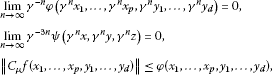(2.1)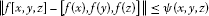(2.2)

for all $\mu \in {\mathbb{T}}^{1}$ and all $x,y,z,{x}_{1},\dots ,{x}_{p},{y}_{1},\dots ,{y}_{d}\in A$, where $\gamma =\frac{p+2d}{2}$. If there exists constant $L<1$ such that

$\phi \left(\gamma x,\dots ,\gamma x\right)\le \gamma L\phi \left(x,\dots ,x\right)$

for all $x\in A$, then there exists a unique ${C}^{\ast }$-ternary algebras homomorphism $H:A\to B$ satisfying

$\parallel f\left(x\right)-H\left(x\right)\parallel \le \frac{1}{\left(1-L\right)2\gamma }\phi \left(x,\dots ,x\right)$
(2.3)

for all $x\in A$.

Proof Let us assume $\mu =1$ and ${x}_{1}=\cdots ={x}_{p}={y}_{1}=\cdots ={y}_{d}=x$ in (2.1). Then we get

$\parallel f\left(\gamma x\right)-\gamma f\left(x\right)\parallel \le \frac{1}{2}\phi \left(x,\dots ,x\right)$
(2.4)

for all $x\in A$. Let $E:=\left\{g:A\to B\right\}$. We introduce a generalized metric on E as follows:

It is easy to show that $\left(E,d\right)$ is a generalized complete metric space.

Now, we consider the mapping $\mathrm{\Lambda }:E\to E$ defined by

Let $g,h\in E$ and let $C\in \left[0,\mathrm{\infty }\right]$ be an arbitrary constant with $d\left(g,h\right)\le C$. From the definition of d, we have

$\parallel g\left(x\right)-h\left(x\right)\parallel \le C\phi \left(x,\dots ,x\right)$

for all $x\in A$. By the assumption and the last inequality, we have

$\parallel \left(\mathrm{\Lambda }g\right)\left(x\right)-\left(\mathrm{\Lambda }h\right)\left(x\right)\parallel =\frac{1}{\gamma }\parallel g\left(\gamma x\right)-h\left(\gamma x\right)\parallel \le \frac{C}{\gamma }\phi \left(\gamma x,\dots ,\gamma x\right)\le CL\phi \left(x,\dots ,x\right)$

for all $x\in A$. So $d\left(\mathrm{\Lambda }g,\mathrm{\Lambda }h\right)\le Ld\left(g,h\right)$ for any $g,h\in E$. It follows from (2.4) that $d\left(\mathrm{\Lambda }f,f\right)\le \frac{1}{2\gamma }$. Therefore according to Theorem 1.2, the sequence $\left\{{\mathrm{\Lambda }}^{n}f\right\}$ converges to a fixed point H of Λ, i.e.,

$H:A\to B,\phantom{\rule{1em}{0ex}}H\left(x\right)=\underset{n\to \mathrm{\infty }}{lim}\left({\mathrm{\Lambda }}^{n}f\right)\left(x\right)=\underset{n\to \mathrm{\infty }}{lim}\frac{1}{{\gamma }^{n}}f\left({\gamma }^{n}x\right)$
(2.5)

and $H\left(\gamma x\right)=\gamma H\left(x\right)$ for all $x\in A$. Also H is the unique fixed point of Λ in the set $E=\left\{g\in E:d\left(f,g\right)<\mathrm{\infty }\right\}$ and

$d\left(H,f\right)\le \frac{1}{1-L}d\left(\mathrm{\Lambda }f,f\right)\le \frac{1}{\left(1-L\right)2\gamma }$

i.e., the inequality (2.3) holds true for all $x\in A$. It follows from the definition of H that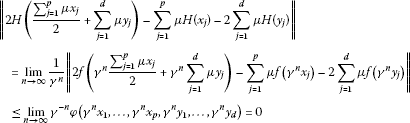for all $\mu \in {\mathbb{T}}^{1}$ and all ${x}_{1},\dots ,{x}_{p},{y}_{1},\dots ,{y}_{d}\in A$. Hence

$2H\left(\frac{{\sum }_{j=1}^{p}\mu {x}_{j}}{2}+\sum _{j=1}^{d}\mu {y}_{j}\right)=\sum _{j=1}^{p}\mu H\left({x}_{j}\right)+2\sum _{j=1}^{d}\mu H\left({y}_{j}\right)$

for all $\mu \in {\mathbb{T}}^{1}$ and all ${x}_{1},\dots ,{x}_{p},{y}_{1},\dots ,{y}_{d}\in A$. So $H\left(\lambda x+\mu y\right)=\lambda H\left(x\right)+\mu H\left(y\right)$ for all $\lambda ,\mu \in {\mathbb{T}}^{1}$ and all $x,y\in A$.

Therefore, by Lemma 2.1, the mapping $H:A\to B$ is $\mathbb{C}$-linear.

It follows from (2.2) and (2.5) that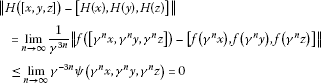for all $x,y,z\in A$. Thus

$H\left(\left[x,y,z\right]\right)=\left[H\left(x\right),H\left(y\right),H\left(z\right)\right]$

for all $x,y,z\in A$. Therefore, the mapping H is a ${C}^{\ast }$-ternary algebras homomorphism.

Now, let $T:A\to B$ be another ${C}^{\ast }$-ternary algebras homomorphism satisfying (2.3). Since $d\left(f,T\right)\le \frac{1}{\left(1-L\right)2\gamma }$ and T is $\mathbb{C}$-linear, we get $T\in {E}^{\prime }$ and $\left(\mathrm{\Lambda }T\right)\left(x\right)=\frac{1}{\gamma }\left(T\gamma x\right)=T\left(x\right)$ for all $x\in A$, i.e., T is a fixed point of Λ. Since H is the unique fixed point of $\mathrm{\Lambda }\in {E}^{\prime }$, we get $H=T$. □

Theorem 2.4 Let $f:A\to B$ be a mapping for which there exist functions $\phi :{A}^{p+d}\to \left[0,\mathrm{\infty }\right)$ and $\psi :{A}^{3}\to \left[0,\mathrm{\infty }\right)$ satisfying (2.1), (2.2),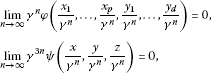for all $x,y,z,{x}_{1},\dots ,{x}_{p},{y}_{1},\dots ,{y}_{d}\in A$, where $\gamma =\frac{p+2d}{2}$. If there exists constant $L<1$ such that

$\phi \left(\frac{1}{\gamma }x,\dots ,\frac{1}{\gamma }x\right)\le \frac{1}{\gamma }L\phi \left(x,\dots ,x\right)$

for all $x\in A$, then there exists a unique ${C}^{\ast }$-ternary algebras homomorphism $H:A\to B$ satisfying

$\parallel f\left(x\right)-H\left(x\right)\parallel \le \frac{1}{\left(1-L\right)2\gamma }\phi \left(x,\dots ,x\right)$

for all $x\in A$.

Proof If we replace x in (2.4) by $\frac{x}{\gamma }$, then we get

$\parallel f\left(x\right)-\gamma f\left(\frac{x}{\gamma }\right)\parallel \le \frac{1}{2}\phi \left(\frac{x}{\gamma },\dots ,\frac{x}{\gamma }\right)\le \frac{L}{2\gamma }\phi \left(x,\dots ,x\right)$
(2.6)

for all $x\in A$. Let $E:=\left\{g:A\to A\right\}$. We introduce a generalized metric on E as follows:

It is easy to show that $\left(E,d\right)$ is a generalized complete metric space.

Now, we consider the mapping $\mathrm{\Lambda }:E\to E$ defined by

Let $g,h\in E$ and let $C\in \left[0,\mathrm{\infty }\right]$ be an arbitrary constant with $d\left(g,h\right)\le C$. From the definition of d, we have

$\parallel g\left(x\right)-h\left(x\right)\parallel \le C\phi \left(x,\dots ,x\right)$

for all $x\in A$. By the assumption and the last inequality, we have

$\parallel \left(\mathrm{\Lambda }g\right)\left(x\right)-\left(\mathrm{\Lambda }h\right)\left(x\right)\parallel =\parallel \gamma g\left(\frac{x}{\gamma }\right)-\gamma h\left(\frac{x}{\gamma }\right)\parallel \le \gamma C\phi \left(\frac{x}{\gamma },\dots ,\frac{x}{\gamma }\right)\le CL\phi \left(x,\dots ,x\right)$

for all $x\in A$, and so $d\left(\mathrm{\Lambda }g,\mathrm{\Lambda }h\right)\le Ld\left(g,h\right)$ for any $g,h\in E$. It follows from (2.6) that $d\left(\mathrm{\Lambda }f,f\right)\le \frac{1}{2\gamma }$. Thus, according to Theorem 1.2, the sequence $\left\{{\mathrm{\Lambda }}^{n}f\right\}$ converges to a fixed point H of Λ, i.e.,

$H:A\to B,\phantom{\rule{1em}{0ex}}H\left(x\right)=\underset{n\to \mathrm{\infty }}{lim}\left({\mathrm{\Lambda }}^{n}f\right)\left(x\right)=\underset{n\to \mathrm{\infty }}{lim}{\gamma }^{n}f\left(\frac{x}{{\gamma }^{n}}\right)$

for all $x\in A$.

The rest of the proof is similar to the proof of Theorem 2.3, and we omit it. □

Corollary 2.5 ()

Let r and θ be nonnegative real numbers such that $r\notin \left[1,3\right]$, and let $f:A\to B$ be a mapping such that

$\parallel {C}_{\mu }f\left({x}_{1},\dots ,{x}_{p},{y}_{1},\dots ,{y}_{d}\right)\parallel \le \theta \left(\sum _{j=1}^{p}{\parallel {x}_{j}\parallel }^{r}+\sum _{j=1}^{d}{\parallel {y}_{j}\parallel }^{r}\right)$
(2.7)

and

$\parallel f\left(\left[x,y,z\right]\right)-\left[f\left(x\right),f\left(y\right),f\left(z\right)\right]\parallel \le \theta \left({\parallel x\parallel }^{r}+{\parallel y\parallel }^{r}+{\parallel z\parallel }^{r}\right)$
(2.8)

for all $\mu \in {\mathbb{T}}^{1}$ and all $x,y,z,{x}_{1},\dots ,{x}_{p},{y}_{1},\dots ,{y}_{d}\in A$. Then there exists a unique ${C}^{\ast }$-ternary algebra homomorphism $H:A\to B$ such that

$\parallel f\left(x\right)-H\left(x\right)\parallel \le \frac{{2}^{r}\left(p+d\right)\theta }{|2{\left(p+2d\right)}^{r}-\left(p+2d\right){2}^{r}|}{\parallel x\parallel }^{r}$
(2.9)

for all $x\in A$.

Proof The proof follows from Theorems 2.3 and 2.4 by taking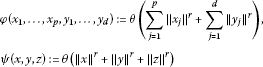for all $\mu \in {\mathbb{T}}^{1}$ and all $x,y,z,{x}_{1},\dots ,{x}_{p},{y}_{1},\dots ,{y}_{d}\in A$. Then we can choose $L={2}^{1-r}{\left(p+2d\right)}^{r-1}$, when $0 and $L=2-{2}^{1-r}{\left(p+2d\right)}^{r-1}$, when $r>3$ and we get the desired results. □

## 3 Superstability of homomorphisms in ${C}^{\ast }$-ternary algebras

Throughout this section, assume that A is a unital ${C}^{\ast }$-ternary algebra with norm $\parallel \cdot \parallel$ and unit e, and that B is a unital ${C}^{\ast }$-ternary algebra with norm $\parallel \cdot \parallel$ and unit ${e}^{\prime }$.

We investigate homomorphisms in ${C}^{\ast }$-ternary algebras associated with the functional equation ${C}_{\mu }f\left({x}_{1},\dots ,{x}_{p},{y}_{1},\dots ,{y}_{d}\right)=0$.

Theorem 3.1 ()

Let $r>1$ (resp., $r<1$) and θ be nonnegative real numbers, and let $f:A\to B$ be a bijective mapping satisfying (2.1) and

$f\left(\left[x,y,z\right]\right)=\left[f\left(x\right),f\left(y\right),f\left(z\right)\right]$

for all $x,y,z\in A$. If ${lim}_{n\to \mathrm{\infty }}\frac{{\left(p+2d\right)}^{n}}{{2}^{n}}f\left(\frac{{2}^{n}e}{{\left(p+2d\right)}^{n}}\right)={e}^{\prime }$ (resp., ${lim}_{n\to \mathrm{\infty }}\frac{{2}^{n}}{{\left(p+2d\right)}^{n}}f\left(\frac{{\left(p+2d\right)}^{n}}{{2}^{n}}e\right)={e}^{\prime }$), then the mapping $f:A\to B$ is a ${C}^{\ast }$-ternary algebra isomorphism.

In the following theorems we have alternative results of Theorem 3.1.

Theorem 3.2 Let $r<1$ and θ be nonnegative real numbers, and let $f:A\to B$ be a mapping satisfying (2.7) and (2.8). If there exist a real number $\lambda >1$ (resp., $0<\lambda <1$) and an element ${x}_{0}\in A$ such that ${lim}_{n\to \mathrm{\infty }}\frac{1}{{\lambda }^{n}}f\left({\lambda }^{n}{x}_{0}\right)={e}^{\prime }$ (resp., ${lim}_{n\to \mathrm{\infty }}{\lambda }^{n}f\left(\frac{{x}_{0}}{{\lambda }^{n}}\right)={e}^{\prime }$), then the mapping $f:A\to B$ is a ${C}^{\ast }$-ternary algebra homomorphism.

Proof By using the proof of Corollary 2.5, there exists a unique ${C}^{\ast }$-ternary algebra homomorphism $H:A\to B$ satisfying (2.9). It follows from (2.9) that

$H\left(x\right)=\underset{n\to \mathrm{\infty }}{lim}\frac{1}{{\lambda }^{n}}f\left({\lambda }^{n}x\right),\phantom{\rule{2em}{0ex}}\left(H\left(x\right)=\underset{n\to \mathrm{\infty }}{lim}{\lambda }^{n}f\left(\frac{x}{{\lambda }^{n}}\right)\right)$

for all $x\in A$ and all real numbers $\lambda >1$ ($0<\lambda <1$). Therefore, by the assumption, we get that $H\left({x}_{0}\right)={e}^{\prime }$.

Let $\lambda >1$ and ${lim}_{n\to \mathrm{\infty }}\frac{1}{{\lambda }^{n}}f\left({\lambda }^{n}{x}_{0}\right)={e}^{\prime }$. It follows from (2.8) that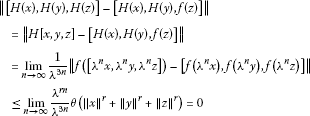for all $x\in A$. So $\left[H\left(x\right),H\left(y\right),H\left(z\right)\right]=\left[H\left(x\right),H\left(y\right),f\left(z\right)\right]$ for all $x,y,z\in A$. Letting $x=y={x}_{0}$ in the last equality, we get $f\left(z\right)=H\left(z\right)$ for all $z\in A$. Similarly, one can show that $H\left(x\right)=f\left(x\right)$ for all $x\in A$ when $0<\lambda <1$ and ${lim}_{n\to \mathrm{\infty }}{\lambda }^{n}f\left(\frac{{x}_{0}}{{\lambda }^{n}}\right)={e}^{\prime }$.

Similarly, one can show the theorem for the case $\lambda >1$.

Therefore, the mapping $f:A\to B$ is a ${C}^{\ast }$-ternary algebra homomorphism. □

Theorem 3.3 Let $r>1$ and θ be nonnegative real numbers, and let $f:A\to B$ be a mapping satisfying (2.7) and (2.8). If there exist a real number $0<\lambda <1$ (resp., $\lambda >1$) and an element ${x}_{0}\in A$ such that ${lim}_{n\to \mathrm{\infty }}\frac{1}{{\lambda }^{n}}f\left({\lambda }^{n}{x}_{0}\right)={e}^{\prime }$ (resp., ${lim}_{n\to \mathrm{\infty }}{\lambda }^{n}f\left(\frac{{x}_{0}}{{\lambda }^{n}}\right)={e}^{\prime }$), then the mapping $f:A\to B$ is a ${C}^{\ast }$-ternary algebra homomorphism.

Proof The proof is similar to the proof of Theorem 3.2 and we omit it. □

## 4 Stability of derivations on ${C}^{\ast }$-ternary algebras

Throughout this section, assume that A is a ${C}^{\ast }$-ternary algebra with norm $\parallel \cdot \parallel$.

Park  proved the Hyers-Ulam stability of derivations on ${C}^{\ast }$-ternary algebras for the functional equation ${C}_{\mu }f\left({x}_{1},\dots ,{x}_{p},{y}_{1},\dots ,{y}_{d}\right)=0$.

For a given mapping $f:A\to A$, let

$\mathbf{D}f\left(x,y,z\right)=f\left(\left[x,y,z\right]\right)-\left[f\left(x\right),y,z\right]-\left[x,f\left(y\right),z\right]-\left[x,y,f\left(z\right)\right]$

for all $x,y,z\in A$.

Theorem 4.1 ()

Let r and θ be nonnegative real numbers such that $r\notin \left[1,3\right]$, and let $f:A\to A$ be a mapping satisfying (2.7) and

$\parallel \mathbf{D}f\left(x,y,z\right)\parallel \le \theta \left({\parallel x\parallel }^{r}+{\parallel y\parallel }^{r}+{\parallel z\parallel }^{r}\right)$

for all $x,y,z\in A$. Then there exists a unique ${C}^{\ast }$-ternary derivation $\delta :A\to A$ such that

$\parallel f\left(x\right)-\delta \left(x\right)\parallel \le \frac{{2}^{r}\left(p+d\right)}{|2{\left(p+2d\right)}^{r}-\left(p+2d\right){2}^{r}|}\theta {\parallel x\parallel }^{r}$

for all $x\in A$.

In the following theorem, we generalize and improve the result in Theorems 4.1.

Theorem 4.2 Let $\phi :{A}^{p+d}\to \left[0,\mathrm{\infty }\right)$ and $\psi :{A}^{3}\to \left[0,\mathrm{\infty }\right)$ be functions such that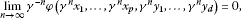(4.1)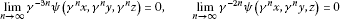(4.2)

for all $x,y,z,{x}_{1},\dots ,{x}_{p},{y}_{1},\dots ,{y}_{d}\in A$, where $\gamma =\frac{p+2d}{2}$. Suppose that $f:A\to A$ is a mapping satisfying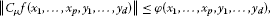(4.3)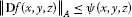(4.4)

for all $\mu \in {\mathbb{T}}^{1}$ and all $x,y,z,{x}_{1},\dots ,{x}_{p},{y}_{1},\dots ,{y}_{d}\in A$. If there exists a constant $L<1$ such that

$\phi \left(\gamma x,\dots ,\gamma x\right)\le \gamma \phi \left(x,\dots ,x\right),$

then the mapping $f:A\to A$ is a ${C}^{\ast }$-ternary derivation.

Proof Let us assume $\mu =1$ and ${x}_{1}=\cdots ={x}_{p}={y}_{1}=\cdots ={y}_{d}=x$ in (4.3). Then we get

$\parallel f\left(\gamma x\right)-\gamma f\left(x\right)\parallel \le \frac{1}{2}\phi \left(x,\dots ,x\right)$
(4.5)

for all $x\in A$. Let $E:=\left\{g:A\to A\right\}$. We introduce a generalized metric on E as follows:

It is easy to show that $\left(E,d\right)$ is a generalized complete metric space.

Now, we consider the mapping $\mathrm{\Lambda }:E\to E$ defined by

Let $g,h\in E$ and let $C\in \left[0,\mathrm{\infty }\right]$ be an arbitrary constant with $d\left(g,h\right)\le C$. From the definition of d, we have

$\parallel g\left(x\right)-h\left(x\right)\parallel \le C\phi \left(x,\dots ,x\right)$

for all $x\in A$. By the assumption and the last inequality, we have

$\parallel \left(\mathrm{\Lambda }g\right)\left(x\right)-\left(\mathrm{\Lambda }h\right)\left(x\right)\parallel =\frac{1}{\gamma }\parallel g\left(\gamma x\right)-h\left(\gamma x\right)\parallel \le \frac{C}{\gamma }\phi \left(\gamma x,\dots ,\gamma x\right)\le CL\phi \left(x,\dots ,x\right)$

for all $x\in A$. Then $d\left(\mathrm{\Lambda }g,\mathrm{\Lambda }h\right)\le Ld\left(g,h\right)$ for any $g,h\in E$. It follows from (2.4) that $d\left(\mathrm{\Lambda }f,f\right)\le \frac{1}{2\gamma }$. Thus according to Theorem 1.2, the sequence $\left\{{\mathrm{\Lambda }}^{n}f\right\}$ converges to a fixed point δ of Λ, i.e.,

$\delta :A\to A,\phantom{\rule{1em}{0ex}}\delta \left(x\right)=\underset{n\to \mathrm{\infty }}{lim}\left({\mathrm{\Lambda }}^{n}f\right)\left(x\right)=\underset{n\to \mathrm{\infty }}{lim}\frac{1}{{\gamma }^{n}}f\left({\gamma }^{n}x\right)$
(4.6)

and $\delta \left(\gamma x\right)=\gamma \delta \left(x\right)$ for all $x\in A$. Also δ is the unique fixed point of Λ in the set $E=\left\{g\in E:d\left(f,g\right)<\mathrm{\infty }\right\}$ and

$d\left(\delta ,f\right)\le \frac{1}{1-L}d\left(\mathrm{\Lambda }f,f\right)\le \frac{1}{\left(1-L\right)2\gamma }$

i.e., the inequality (2.3) holds true for all $x\in A$. It follows from the definition of δ, (4.1), (4.3), and (4.6) that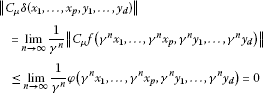for all $\mu \in {\mathbb{T}}^{1}$ and all $x,y,z,{x}_{1},\dots ,{x}_{p},{y}_{1},\dots ,{y}_{d}\in A$. Hence,

$2\delta \left(\frac{{\sum }_{j=1}^{p}\mu {x}_{j}}{2}+\sum _{j=1}^{d}\mu {y}_{j}\right)=\sum _{j=1}^{p}\mu \delta \left({x}_{j}\right)+2\sum _{j=1}^{d}\mu \delta \left({y}_{j}\right)$

for all $\mu \in {\mathbb{T}}^{1}$ and all ${x}_{1},\dots ,{x}_{p},{y}_{1},\dots ,{y}_{d}\in A$. So $\delta \left(\lambda x+\mu y\right)=\lambda \delta \left(x\right)+\mu \delta \left(y\right)$ for all $\lambda ,\mu \in {\mathbb{T}}^{1}$ and all $x,y\in A$.

Therefore, by Lemma 2.1 the mapping $\delta :A\to A$ is $\mathbb{C}$-linear.

It follows from (4.2) and (4.4) that

$\parallel \mathbf{D}\delta \left(x,y,z\right)\parallel =\underset{n\to \mathrm{\infty }}{lim}\frac{1}{{\gamma }^{3n}}\parallel \mathbf{D}f\left({\gamma }^{n}x,{\gamma }^{n}y,{\gamma }^{n}z\right)\parallel \le \underset{n\to \mathrm{\infty }}{lim}\frac{1}{{\gamma }^{3n}}\psi \left({\gamma }^{n}x,{\gamma }^{n}y,{\gamma }^{n}z\right)=0$

for all $x,y,z\in A$. Hence

$\delta \left(\left[x,y,z\right]\right)=\left[\delta \left(x\right),y,z\right]+\left[x,\delta \left(y\right),z\right]+\left[x,y,\delta \left(z\right)\right]$
(4.7)

for all $x,y,z\in A$. So the mapping $\delta :A\to A$ is a ${C}^{\ast }$-ternary derivation.

It follows from (4.2) and (4.4)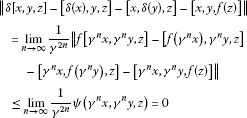for all $x,y,z\in A$. Thus

$\delta \left[x,y,z\right]=\left[\delta \left(x\right),y,z\right]+\left[x,\delta \left(y\right),z\right]+\left[x,y,f\left(z\right)\right]$
(4.8)

for all $x,y,z\in A$. Hence, we get from (4.7) and (4.8) that

$\left[x,y,\delta \left(z\right)\right]=\left[x,y,f\left(z\right)\right]$
(4.9)

for all $x,y,z\in A$. Letting $x=y=f\left(z\right)-\delta \left(z\right)$ in (4.9), we get

${\parallel f\left(z\right)-\delta \left(z\right)\parallel }^{3}=\parallel \left[f\left(z\right)-\delta \left(z\right),f\left(z\right)-\delta \left(z\right),f\left(z\right)-\delta \left(z\right)\right]\parallel =0$

for all $z\in A$. Hence, $f\left(z\right)=\delta \left(z\right)$ for all $z\in A$. So the mapping $f:A\to A$ is a ${C}^{\ast }$-ternary derivation, as desired. □

Corollary 4.3 Let $r<1$, $s<2$ and θ be nonnegative real numbers, and let $f:A\to A$ be a mapping satisfying (2.7) and

${\parallel \mathbf{D}f\left(x,y,z\right)\parallel }_{A}\le \theta \left({\parallel x\parallel }_{A}^{s}+{\parallel y\parallel }_{A}^{s}+{\parallel z\parallel }_{A}^{s}\right)$

for all $x,y,z\in A$. Then the mapping $f:A\to A$ is a ${C}^{\ast }$-ternary derivation.

Proof Defining

$\phi \left({x}_{1},\dots ,{x}_{p},{y}_{1},\dots ,{y}_{d}\right)=\theta \left(\sum _{j=1}^{p}{\parallel {x}_{j}\parallel }_{A}^{r}+\sum _{j=1}^{d}{\parallel {y}_{j}\parallel }_{A}^{r}\right)$

and

$\psi \left(x,y,z\right)=\theta \left({\parallel x\parallel }_{A}^{s}+{\parallel y\parallel }_{A}^{s}+{\parallel z\parallel }_{A}^{s}\right)$

for all $x,y,z,{x}_{1},\dots ,{x}_{p},{y}_{1},\dots ,{y}_{d}\in A$, and applying Theorem 4.2, we get the desired result. □

Theorem 4.4 Let $\phi :{A}^{p+d}\to \left[0,\mathrm{\infty }\right)$ and $\psi :{A}^{3}\to \left[0,\mathrm{\infty }\right)$ be functions such that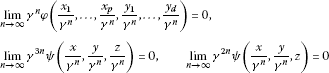for all $x,y,z,{x}_{1},\dots ,{x}_{p},{y}_{1},\dots ,{y}_{d}\in A$ where $\gamma =\frac{p+2d}{2}$. Suppose that $f:A\to A$ is a mapping satisfying (4.3) and (4.4). If there exists a constant $L<1$ such that

$\phi \left(\frac{x}{\gamma },\dots ,\frac{x}{\gamma }\right)\le \frac{L}{\gamma }\phi \left(x,\dots ,x\right),$

then the mapping $f:A\to A$ is a ${C}^{\ast }$-ternary derivation.

Proof If we replace x in (4.5) by $\frac{x}{\gamma }$, then we get

${\parallel f\left(x\right)-\gamma f\left(\frac{x}{\gamma }\right)\parallel }_{A}\le \frac{1}{2}\phi \left(\frac{x}{\gamma },\dots ,\frac{x}{\gamma }\right)$

for all $x\in A$. Let $E:=\left\{g:A\to A\right\}$. We introduce a generalized metric on E as follows:

It is easy to show that $\left(E,d\right)$ is a generalized complete metric space.

Now, we consider the mapping $\mathrm{\Lambda }:E\to E$ defined by

Let $g,h\in E$ and let $C\in \left[0,\mathrm{\infty }\right]$ be an arbitrary constant with $d\left(g,h\right)\le C$. From the definition of d, we have

$\parallel g\left(x\right)-h\left(x\right)\parallel \le C\phi \left(x,\dots ,x\right)$

for all $x\in A$. By the assumption and last inequality, we have

$\parallel \left(\mathrm{\Lambda }g\right)\left(x\right)-\left(\mathrm{\Lambda }h\right)\left(x\right)\parallel =\parallel \gamma g\left(\frac{x}{\gamma }\right)-\gamma h\left(\frac{x}{\gamma }\right)\parallel \le \gamma C\phi \left(\frac{x}{\gamma },\dots ,\frac{x}{\gamma }\right)\le CL\phi \left(x,\dots ,x\right)$

for all $x\in A$. Then $d\left(\mathrm{\Lambda }g,\mathrm{\Lambda }h\right)\le Ld\left(g,h\right)$ for any $g,h\in E$. It follows from (4.5) that $d\left(\mathrm{\Lambda }f,f\right)\le \frac{1}{2\gamma }$. Therefore according to Theorem 1.2, the sequence $\left\{{\mathrm{\Lambda }}^{n}f\right\}$ converges to a fixed point δ of Λ, i.e.,

$\delta :A\to A,\phantom{\rule{1em}{0ex}}\delta \left(x\right)=\underset{n\to \mathrm{\infty }}{lim}\left({\mathrm{\Lambda }}^{n}f\right)\left(x\right)=\underset{n\to \mathrm{\infty }}{lim}{\gamma }^{n}f\left(\frac{x}{{\gamma }^{n}}\right)$

and $\delta \left(\gamma x\right)=\gamma \delta \left(x\right)$ for all $x\in A$.

The rest of the proof is similar to the proof of Theorem 4.2, and we omit it. □

## References

1. Ulam SM: A Collection of the Mathematical Problems. Interscience, New York; 1960.

2. Hyers DH: On the stability of the linear functional equation. Proc. Natl. Acad. Sci. USA 1941, 27: 222–224. 10.1073/pnas.27.4.222

3. Aoki T: On the stability of the linear transformation in Banach spaces. J. Math. Soc. Jpn. 1950, 2: 64–66. 10.2969/jmsj/00210064

4. Rassias TM: On the stability of the linear mapping in Banach spaces. Proc. Am. Math. Soc. 1978, 72: 297–300. 10.1090/S0002-9939-1978-0507327-1

5. Rassias TM: Problem 16; 2. Report of the 27th international symp. on functional equations. Aequ. Math. 1990, 39: 292–293.

6. Gajda Z: On stability of additive mappings. Int. J. Math. Math. Sci. 1991, 14: 431–434. 10.1155/S016117129100056X

7. Rassias TM, Šemrl P: On the behaviour of mappings which do not satisfy Hyers-Ulam stability. Proc. Am. Math. Soc. 1992, 114: 989–993. 10.1090/S0002-9939-1992-1059634-1

8. Gǎvruta P: A generalization of the Hyers-Ulam-Rassias stability of approximately additive mappings. J. Math. Anal. Appl. 1994, 184: 431–436. 10.1006/jmaa.1994.1211

9. Jung S: On the Hyers-Ulam-Rassias stability of approximately additive mappings. J. Math. Anal. Appl. 1996, 204: 221–226. 10.1006/jmaa.1996.0433

10. Czerwik P: Functional Equations and Inequalities in Several Variables. World Scientific, Singapore; 2002.

11. Hyers DH, Isac G, Rassias TM: Stability of Functional Equations in Several Variables. Birkhäuser, Basel; 1998.

12. Amyari M, Moslehian MS: Approximately ternary semigroup homomorphisms. Lett. Math. Phys. 2006, 77: 1–9. 10.1007/s11005-005-0042-6

13. Bazunova N, Borowiec A, Kerner R: Universal differential calculus on ternary algebras. Lett. Math. Phys. 2004, 67: 195–206.

14. Zettl H: A characterization of ternary rings of operators. Adv. Math. 1983, 48: 117–143. 10.1016/0001-8708(83)90083-X

15. Moslehian MS:Almost derivations on ${C}^{\ast }$-ternary rings. Bull. Belg. Math. Soc. Simon Stevin 2007, 14: 135–142.

16. Kerner, R: Ternary algebraic structures and their applications in physics. Preprint

17. Vainerman L, Kerner R: On special classes of n -algebras. J. Math. Phys. 1996, 37: 2553–2565. 10.1063/1.531526

18. Abramov V, Kerner R, Le Roy B:Hypersymmetry: a ${\mathbb{Z}}_{3}$-graded generalization of supersymmetry. J. Math. Phys. 1997, 38: 1650–1669. 10.1063/1.531821

19. Park C:Isomorphisms between ${C}^{\ast }$-ternary algebras. J. Math. Phys. 2006., 47(10): Article ID 103512

20. Park C:Homomorphisms between Poisson $J{C}^{\ast }$-algebras. Bull. Braz. Math. Soc. 2005, 36: 79–97. 10.1007/s00574-005-0029-z

## Acknowledgements

The authors are grateful to the reviewers for their valuable comments and suggestions.

## Author information

Authors

### Corresponding author

Correspondence to Choonkil Park.

### Competing interests

The authors declare that they have no competing interests.

### Authors’ contributions

All authors carried out the proof. All authors conceived of the study, and participated in its design and coordination. All authors read and approved the final manuscript.

## Rights and permissions

Open Access This article is distributed under the terms of the Creative Commons Attribution 2.0 International License ( https://creativecommons.org/licenses/by/2.0 ), which permits unrestricted use, distribution, and reproduction in any medium, provided the original work is properly cited.

Reprints and Permissions

Kenari, H., Saadati, R. & Park, C. Homomorphisms and derivations in ${C}^{\ast }$-ternary algebras via fixed point method. Adv Differ Equ 2012, 137 (2012). https://doi.org/10.1186/1687-1847-2012-137

• Accepted:

• Published:

• DOI: https://doi.org/10.1186/1687-1847-2012-137

### Keywords

• ${C}^{\ast }$-ternary algebra isomorphism
• generalized Cauchy-Jensen functional equation
• Hyers-Ulam stability
• ${C}^{\ast }$-ternary derivation
• fixed-point method